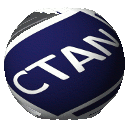# mismath - Miscellaneous mathematical macros

## Presentation

The package provides some mathematical macros to typeset:

• mathematical constants e, i, pi in upright shape (automatically) as recommended by ISO 80000-2,
• vectors with beautiful arrow and adjusted norm,
• some standard operator names,
• improved spacings in mathematical formulas,
• systems of equations and small matrices,
• displaymath in double columns for long calculations.

## Installation

• run on mismath.ins, you obtain the file mismath.sty,
• if then you run pdf on mismath.dtx you get the file mismath.pdf which is also in the archive,
• put the files mismath.sty and mismath.pdf in your Directory Structure.

## Author

Antoine Missier

Email: antoine.missier@ac-toulouse.fr

Released under the Project Public License v1.3 or later. See http://www.latex-project.org/lppl.txt

## mismath – Miscellaneous mathematical macros

The package provides some mathematical macros to typeset:

• mathematical constants e, i, pi in upright shape (automatically) as recommended by ISO 80000-2,
• vectors with beautiful arrows and adjusted norm,
• some standard operator names,
• improved spacings in mathematical formulas,
• systems of equations and small matrices,
• displaymath in double columns for long calculations.

 Package mismath Version 1.7 2019-12-27 Licenses The LaTeX Project Public License 1.3 Copyright 2019 Antoine Missier Maintainer Antoine Missier Contained in TeX Live as mismathMiKTeX as mismath Topics Maths